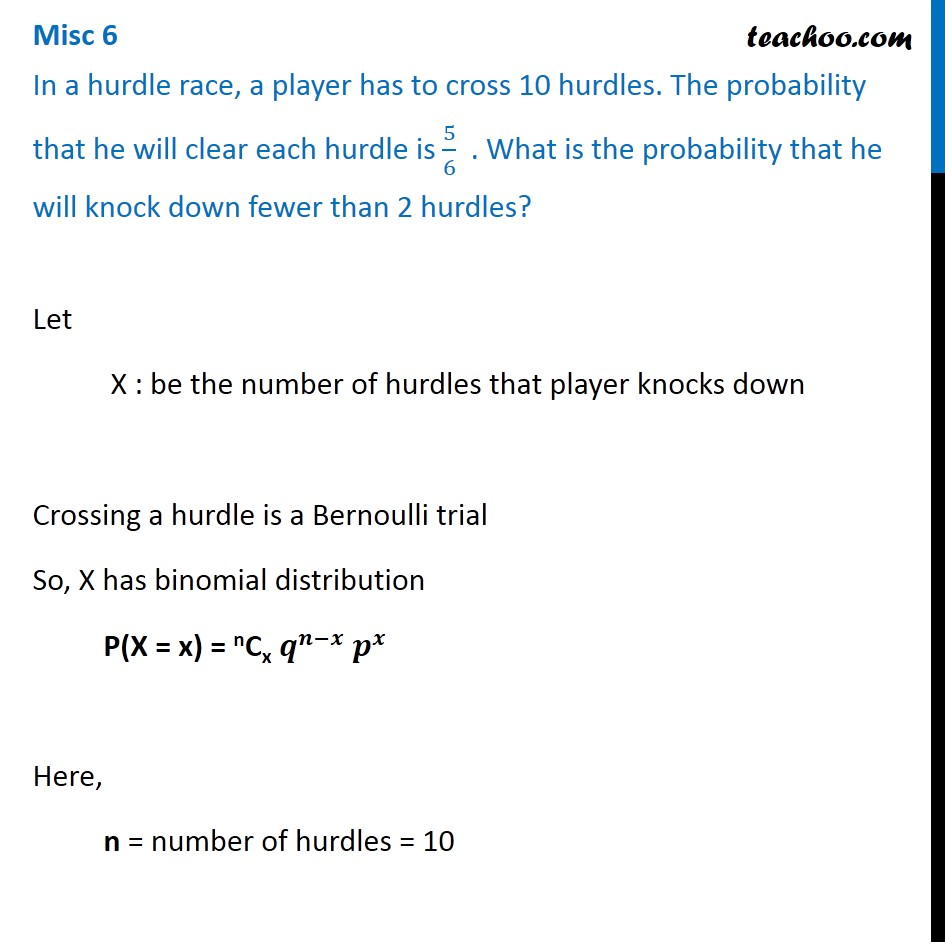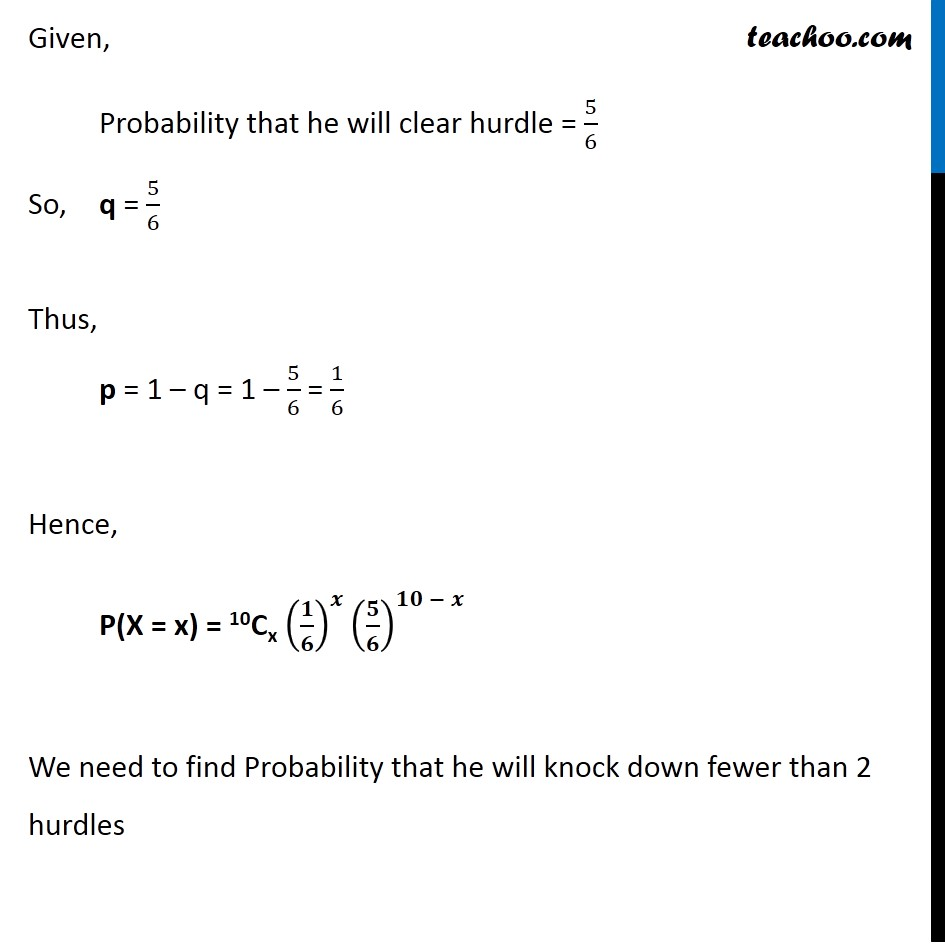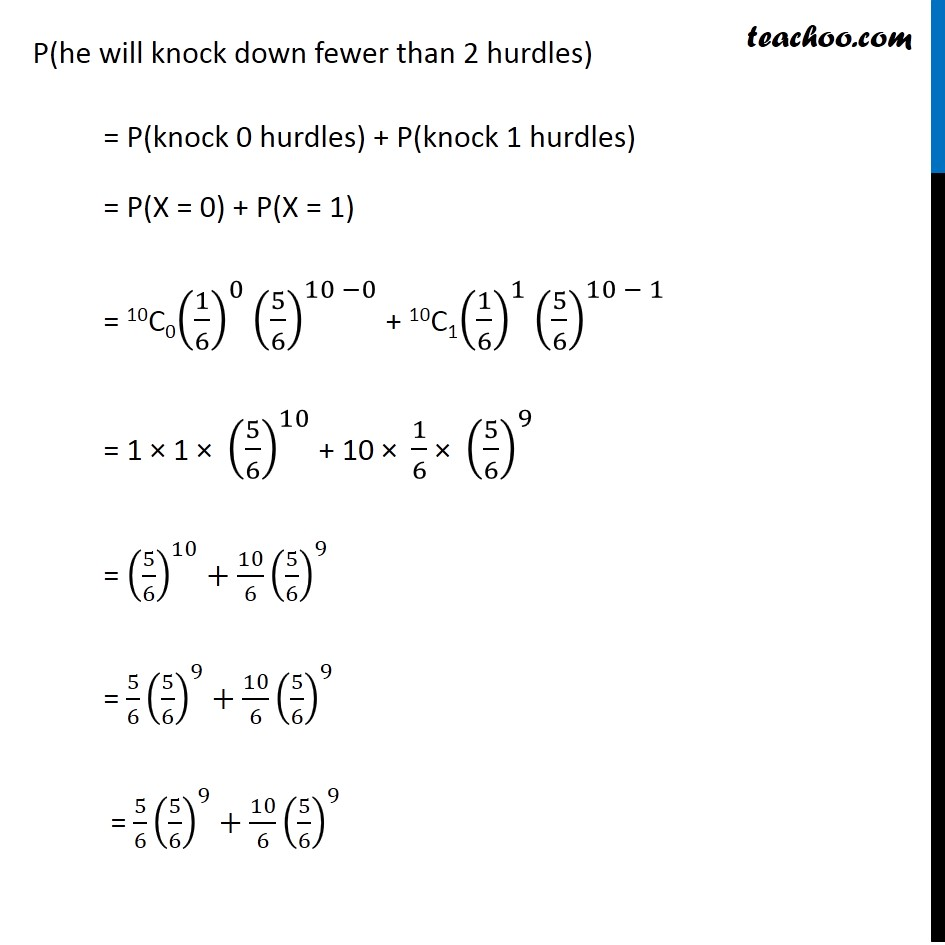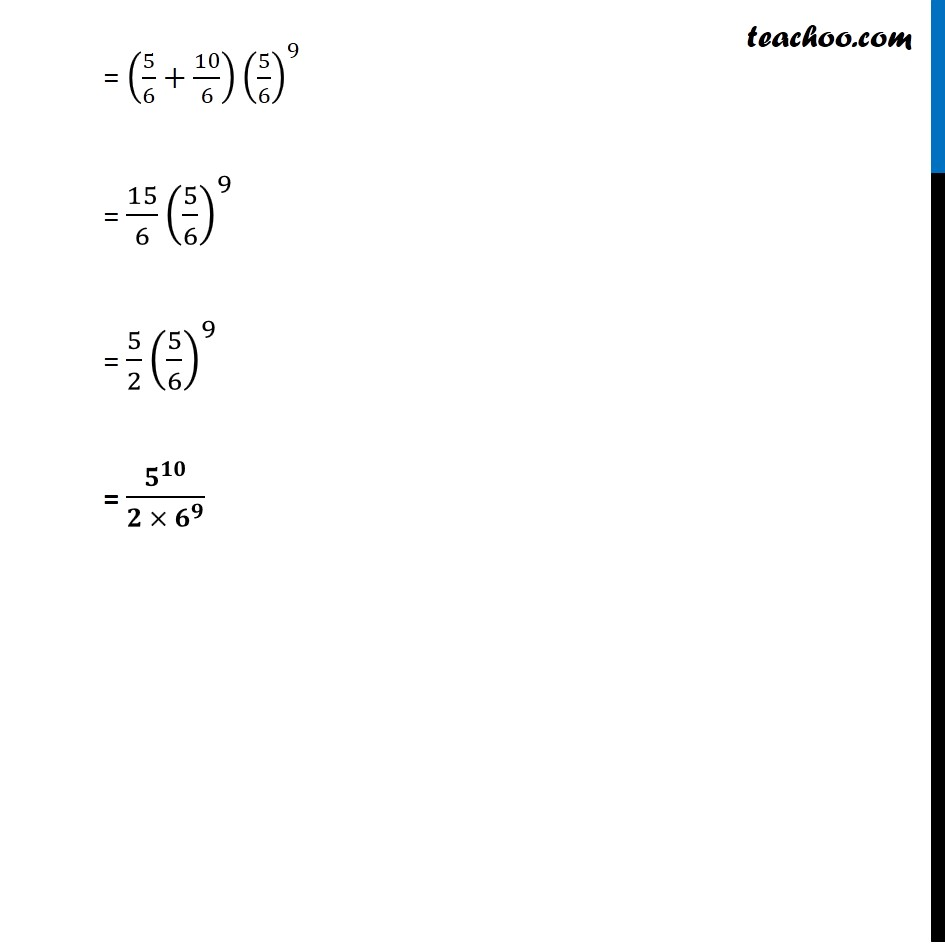Binomial Distribution

Chapter 13 Class 12 Probability
Concept wiseLearn in your speed, with individual attention - Teachoo Maths 1-on-1 Class

### Transcript

Question 2 In a hurdle race, a player has to cross 10 hurdles. The probability that he will clear each hurdle is 5/6 . What is the probability that he will knock down fewer than 2 hurdles?Let X : be the number of hurdles that player knocks down Crossing a hurdle is a Bernoulli trial So, X has binomial distribution P(X = x) = nCx 𝒒^(𝒏−𝒙) 𝒑^𝒙 Here, n = number of hurdles = 10 Given, Probability that he will clear hurdle = 5/6 So, q = 5/6 Thus, p = 1 – q = 1 – 5/6 = 1/6 Hence, P(X = x) = 10Cx (𝟏/𝟔)^𝒙 (𝟓/𝟔)^(𝟏𝟎 − 𝒙) We need to find Probability that he will knock down fewer than 2 hurdles P(he will knock down fewer than 2 hurdles) = P(knock 0 hurdles) + P(knock 1 hurdles) = P(X = 0) + P(X = 1) = 10C0(1/6)^0 (5/6)^(10 −0) + 10C1(1/6)^1 (5/6)^(10 − 1) = 1 × 1 × (5/6)^10 + 10 × 1/6 × (5/6)^9 = (5/6)^10+10/6 (5/6)^9 = 5/6 (5/6)^9+10/6 (5/6)^9 = 5/6 (5/6)^9+10/6 (5/6)^9 = (5/6+10/6) (5/6)^9 = 15/6 (5/6)^9 = 5/2 (5/6)^9 = 𝟓^𝟏𝟎/(𝟐 × 𝟔^𝟗 )